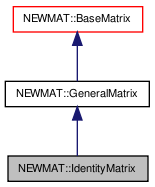# NEWMAT::IdentityMatrix Class Reference

`#include <newmat.h>`

Inheritance diagram for NEWMAT::IdentityMatrix:[legend]

## Detailed Description

Definition at line 1189 of file newmat.h.

List of all members.

## Public Member Functions

IdentityMatrix ()
~IdentityMatrix ()
IdentityMatrix (ArrayLengthSpecifier n)
IdentityMatrix (const IdentityMatrix &gm)
IdentityMatrix (const BaseMatrix &)
void operator= (const BaseMatrix &)
IdentityMatrixoperator= (const IdentityMatrix &m)
IdentityMatrixoperator= (Real f)
MatrixType Type () const
LogAndSign LogDeterminant () const
Real Trace () const
Real SumSquare () const
Real SumAbsoluteValue () const
Real Sum () const
void GetRow (MatrixRowCol &)
void GetCol (MatrixRowCol &)
void GetCol (MatrixColX &)
void NextRow (MatrixRowCol &)
void NextCol (MatrixRowCol &)
void NextCol (MatrixColX &)
GeneralMatrixMakeSolver ()
void Solver (MatrixColX &, const MatrixColX &)
GeneralMatrixTranspose (TransposedMatrix *, MatrixType)
void ReSize (int n)
void ReSize (const GeneralMatrix &A)
MatrixBandWidth BandWidth () const
void swap (IdentityMatrix &gm)

## Private Member Functions

GeneralMatrixImage () const

## Constructor & Destructor Documentation

 NEWMAT::IdentityMatrix::IdentityMatrix ( )

Definition at line 1193 of file newmat.h.

Referenced by Image().

 NEWMAT::IdentityMatrix::~IdentityMatrix ( )

Definition at line 1194 of file newmat.h.

 NEWMAT::IdentityMatrix::IdentityMatrix ( ArrayLengthSpecifier n )

Definition at line 1195 of file newmat.h.

 NEWMAT::IdentityMatrix::IdentityMatrix ( const IdentityMatrix & gm )

Definition at line 1197 of file newmat.h.

 NEWMAT::IdentityMatrix::IdentityMatrix ( const BaseMatrix & M )

Definition at line 153 of file newmat4.cpp.

## Member Function Documentation

 MatrixBandWidth NEWMAT::IdentityMatrix::BandWidth ( ) const` [virtual]`

Reimplemented from NEWMAT::BaseMatrix.

Definition at line 446 of file newmat4.cpp.

 void NEWMAT::IdentityMatrix::GetCol ( MatrixColX & mrc ) ` [virtual]`

Implements NEWMAT::GeneralMatrix.

Definition at line 801 of file newmat3.cpp.

 void NEWMAT::IdentityMatrix::GetCol ( MatrixRowCol & mrc ) ` [virtual]`

Implements NEWMAT::GeneralMatrix.

Definition at line 792 of file newmat3.cpp.

 void NEWMAT::IdentityMatrix::GetRow ( MatrixRowCol & mrc ) ` [virtual]`

Implements NEWMAT::GeneralMatrix.

Definition at line 786 of file newmat3.cpp.

 GeneralMatrix * NEWMAT::IdentityMatrix::Image ( ) const` [private, virtual]`

Reimplemented from NEWMAT::GeneralMatrix.

Definition at line 844 of file newmat4.cpp.

 LogAndSign NEWMAT::IdentityMatrix::LogDeterminant ( ) const` [virtual]`

Reimplemented from NEWMAT::GeneralMatrix.

Definition at line 676 of file newmat8.cpp.

 GeneralMatrix* NEWMAT::IdentityMatrix::MakeSolver ( ) ` [virtual]`

Reimplemented from NEWMAT::GeneralMatrix.

Definition at line 1215 of file newmat.h.

 void NEWMAT::IdentityMatrix::NextCol ( MatrixColX & mrc ) ` [virtual]`

Reimplemented from NEWMAT::GeneralMatrix.

Definition at line 812 of file newmat3.cpp.

 void NEWMAT::IdentityMatrix::NextCol ( MatrixRowCol & mrc ) ` [virtual]`

Reimplemented from NEWMAT::GeneralMatrix.

Definition at line 810 of file newmat3.cpp.

 void NEWMAT::IdentityMatrix::NextRow ( MatrixRowCol & mrc ) ` [virtual]`

Reimplemented from NEWMAT::GeneralMatrix.

Definition at line 808 of file newmat3.cpp.

 IdentityMatrix& NEWMAT::IdentityMatrix::operator= ( Real f )

Reimplemented from NEWMAT::GeneralMatrix.

Definition at line 1201 of file newmat.h.

 IdentityMatrix& NEWMAT::IdentityMatrix::operator= ( const IdentityMatrix & m )

Definition at line 1200 of file newmat.h.

 void NEWMAT::IdentityMatrix::operator= ( const BaseMatrix & X )

Definition at line 415 of file newmat6.cpp.

Referenced by operator=().

 void NEWMAT::IdentityMatrix::ReSize ( const GeneralMatrix & A ) ` [virtual]`

Reimplemented from NEWMAT::GeneralMatrix.

Definition at line 368 of file newmat4.cpp.

 void NEWMAT::IdentityMatrix::ReSize ( int n )

Definition at line 292 of file newmat4.cpp.

Referenced by ReSize().

 void NEWMAT::IdentityMatrix::Solver ( MatrixColX & mrc, const MatrixColX & mrc1 ) ` [virtual]`

Reimplemented from NEWMAT::GeneralMatrix.

Definition at line 514 of file newmat2.cpp.

 Real NEWMAT::IdentityMatrix::Sum ( ) const` [virtual]`

Reimplemented from NEWMAT::GeneralMatrix.

Definition at line 1208 of file newmat.h.

 Real NEWMAT::IdentityMatrix::SumAbsoluteValue ( ) const` [virtual]`

Reimplemented from NEWMAT::GeneralMatrix.

Definition at line 412 of file newmat8.cpp.

 Real NEWMAT::IdentityMatrix::SumSquare ( ) const` [virtual]`

Reimplemented from NEWMAT::GeneralMatrix.

Definition at line 428 of file newmat8.cpp.

 void NEWMAT::IdentityMatrix::swap ( IdentityMatrix & gm )

Definition at line 1222 of file newmat.h.

Referenced by NEWMAT::swap().

 Real NEWMAT::IdentityMatrix::Trace ( ) const` [virtual]`

Reimplemented from NEWMAT::BaseMatrix.

Definition at line 604 of file newmat8.cpp.

Referenced by Sum(), and SumAbsoluteValue().

 GeneralMatrix * NEWMAT::IdentityMatrix::Transpose ( TransposedMatrix * , MatrixType mt ) ` [virtual]`

Reimplemented from NEWMAT::GeneralMatrix.

Definition at line 76 of file newmat5.cpp.

 MatrixType NEWMAT::IdentityMatrix::Type ( ) const` [virtual]`

Implements NEWMAT::GeneralMatrix.

Definition at line 440 of file newmat4.cpp.

The documentation for this class was generated from the following files:

 newmat11b Generated Mon May 9 04:54:19 2016 by Doxygen 1.6.3